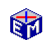The Simple Keynesian Model

The Simple Keynesian Model, which is also known as the Keynesian Cross, emphasizes one basic point.  That point is that a decrease in aggregate demand can lead to a stable equilibrium with substantial unemployment.

The Simple Keynesian Model application first explains the roles of consumption and investment and then explains the accounting identity Y = C + I + G.  Together, these elements determine the equilibrium level of output.

The policy analysis experiments study the effects of animal spirits and fiscal policy.  The numerical results illustrate the calculation of a fiscal policy multiplier.

A concluding experiment extends the model to make investment a function of the interest rate.  Graphing the shifts in investment caused by changes in interest rates then reveals a simple version of the IS curve found in an IS/LM analysis.Model LinkThe Simple Keynesian Model
Printable PDF Exercises

The Simple Keynesian Model is important not so much for its ability to capture the details of recessions, but for its ability to demonstrate the possibility of a stable equilibrium at less than full employment.  While the real wage rate adjusts in the Classical Model to move the economy to full employment, the real wage rate does not appear in the Simple Keynesian Model and equilibrium is achieved by adjustments in aggregate demand, which equals aggregate income.  The equilibrium aggregate income need not imply full employment.

Animal Spirits?

The 2001 U.S. recession could fit the Simple Keynesian Model.  The dot-com meltdown and the 9/11 shock had psychological as well as economic impacts.  Could 2001 be the recession that is due to a failure of animal spirits?

"...most, probably, of our decisions to do something positive, the full consequences of which will be drawn out over many days to come, can only be taken as a result of animal spirits--of a spontaneous urge to action rather than inaction, and not as the outcome of a weighted average of quantitative benefits multiplied by quantitative probabilities...if the animal spirits are dimmed and the spontaneous optimism falters... enterprise will fade and die," - J.M Keynes: The General Theory of Employment, Interest, and Money.

Classic Economic Models

Macroeconomics

Introduction
Overview of Macro Models

Microeconomics

Introduction
Overview of Micro Models

Resources